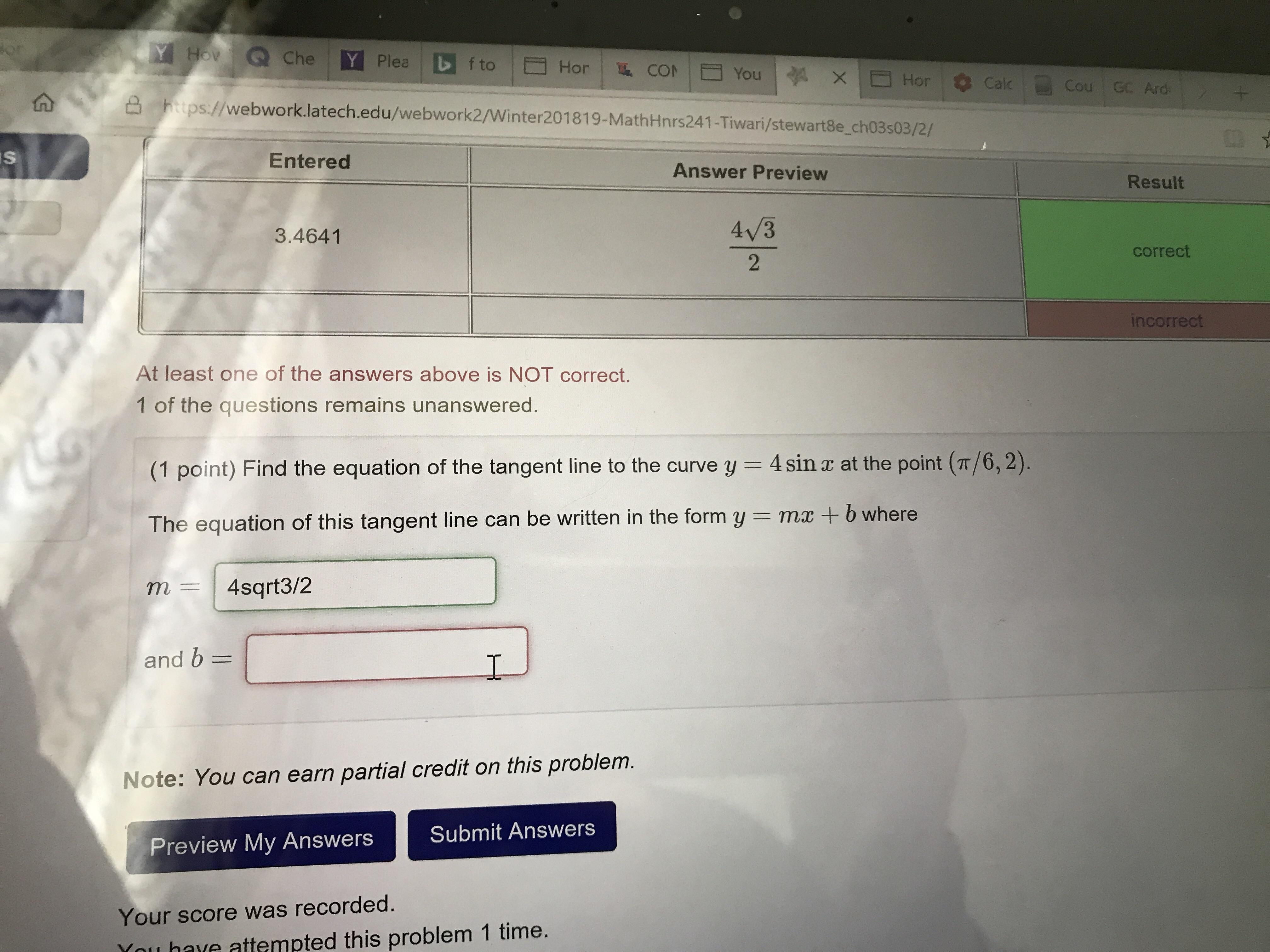# Ho Q Che ps//webwork.latech.edu/webwork2/Winter201819-MathHłnrs241-Tiwari/stewart8e_cho3503/2/ Entered Answer Preview Result 4/3 2 3.4641 correct ncorrec At least one of the answers above is NOT correct. 1 of the questions remains unanswered (1 point) Find the equation of the tangent line to the curve y = 4 sinx at the point (n/6,2). The equation of this tangent line can be written in the form y m+b where m4sqrt3/2 and b Note: You can earn partial credit on this problem. Preview My Answers Submit Answers Your score was recorded. Ynu have attempted this problem 1 time.

Questionhelp_outlineImage TranscriptioncloseHo Q Che ps//webwork.latech.edu/webwork2/Winter201819-MathHłnrs241-Tiwari/stewart8e_cho3503/2/ Entered Answer Preview Result 4/3 2 3.4641 correct ncorrec At least one of the answers above is NOT correct. 1 of the questions remains unanswered (1 point) Find the equation of the tangent line to the curve y = 4 sinx at the point (n/6,2). The equation of this tangent line can be written in the form y m+b where m4sqrt3/2 and b Note: You can earn partial credit on this problem. Preview My Answers Submit Answers Your score was recorded. Ynu have attempted this problem 1 time. fullscreen